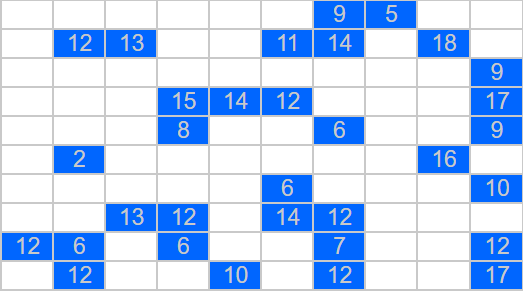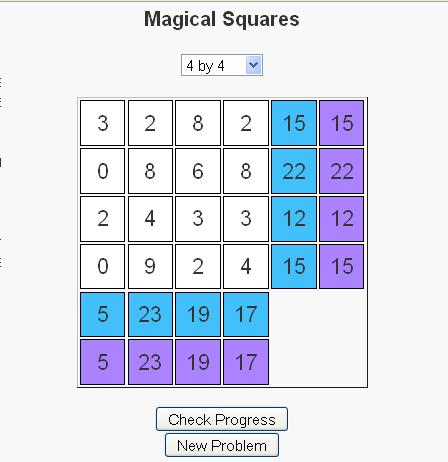# Range Puzzles

Range PuzzlesI have always liked puzzles. I really enjoy discovering a new puzzle. Sometimes when I have discovered a new puzzle, I enjoy it so much that I can’t seem to do enough of them. In such cases, I quickly run out of these puzzles to do and find myself looking for either a new puzzle or a way to generate them on my own.

Such was the case when I discovered the “Range Puzzles”. The rules are simple:

Every cell is marked either blue or gray
No two gray cells can be next to one another
The grid must be a connected (i.e. there is always a path from every cell to every other cell using horizontal and vertical connections.
Some cells have a number inside. This indicates the number of cells that can be viewed (horizontally and vertically in both directions) by this cell, including the cell itself.

To try out these puzzles, visit Range Puzzles at LEARNINGlover.com

# Magical Squares Game

Whether introduced as children in elementary school, as adults in the workplace, or somewhere in between, the concept of magic squares has fascinated people for centuries; The Wikipedia article has discoveries of magic squares dating back to 650 B.C. in China.

Magic Squares of size n (for n >= 3) are n by n grids where the numbers 1, 2, …, n2 are specially arranged such that the sum of each row, column, and diagonal all sum to the same number. Below are two examples of magic squares of size 3 and 4.

 8 3 4 1 5 9 6 2 7
 4 1 16 13 15 11 2 6 10 14 7 3 5 8 9 12

Notice first, that in the first square all the numbers 1, 2, 3, .., 9 are used in the square. Likewise, the numbers 1, 2, …, 15, 16 are used in the second square. Second notice that each row, column and diagonal sums to 15 in the first square and 34 in the second square.

I have recently published a puzzle that is based on the concept of magic squares. There are some slight differences though.
– First, instead of using the numbers 1, 2, …, n2 a random set of numbers are generated.
– Second, the rows and columns each have a desired sum that we would like the numbers in the row/column to sum to.These two changes allow for a puzzle concept to be formulated based on the magic square concept. Users take turns swapping elements until the numbers in each row and column sum to the number in their goal cell, which is located in the last column or row of the grid. Above is an image of a solved puzzle.

Users can determine their progress the numbers in the next-to-last column, which tells the current sum of the numbers in that respective row or column.

To swap two numbers, first click on a cell with one of the two numbers in it. The cell should then turn red. Then click on the cell with the other number in it and the numbers should swap. If you click on the same cell twice no action should take place (except for the cell to turn red and then blank again).

Take a moment to check out the puzzles and let me know what you think.

# Nonogram PuzzlesA friend introduced me to a type of puzzles called Nonograms and I enjoyed them so much that I wrote a script that automatically generates these puzzles.

So have fun with these and let me know what you think.

Other Blogs that have covered this topic:
MINIGAMESCLUB